# 19 Passing Data between pages Forms Sessions Query

• Slides: 40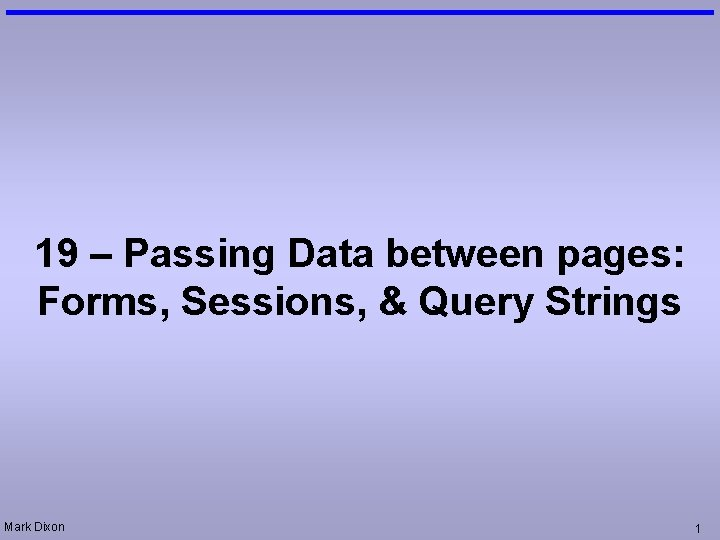19 – Passing Data between pages: Forms, Sessions, & Query Strings Mark Dixon 1Session Aims & Objectives • Aims – To introduce the fundamental ideas involved in passing data between pages – Highlight modular design techniques, and demonstrate them in ASP • Objectives, by end of this week’s sessions, you should be able to: – pass data between pages, using: • Self Posting • Query Strings • Session Variables – use procedures, functions, parameters, and modules (shared VB script files) in ASP Mark Dixon 2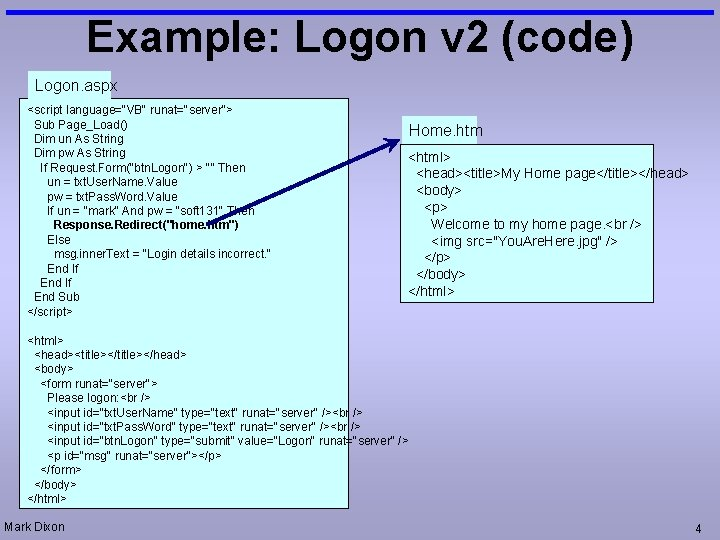Example: Logon v 2 (code) Logon. aspx <script language="VB" runat="server"> Sub Page_Load() Dim un As String Dim pw As String If Request. Form("btn. Logon") > "" Then un = txt. User. Name. Value pw = txt. Pass. Word. Value If un = "mark" And pw = "soft 131" Then Response. Redirect("home. htm") Else msg. inner. Text = "Login details incorrect. " End If End Sub </script> Home. htm <html> <head><title>My Home page</title></head> <body> <p> Welcome to my home page. <img src="You. Are. Here. jpg" /> </p> </body> </html> <head><title></head> <body> <form runat="server"> Please logon: <input id="txt. User. Name" type="text" runat="server" /> <input id="txt. Pass. Word" type="text" runat="server" /> <input id="btn. Logon" type="submit" value="Logon" runat="server" /> <p id="msg" runat="server"></p> </form> </body> </html> Mark Dixon 4Example: Logon (Fixed Problem) • View Source – shows client-side script: No server-side code Mark Dixon 5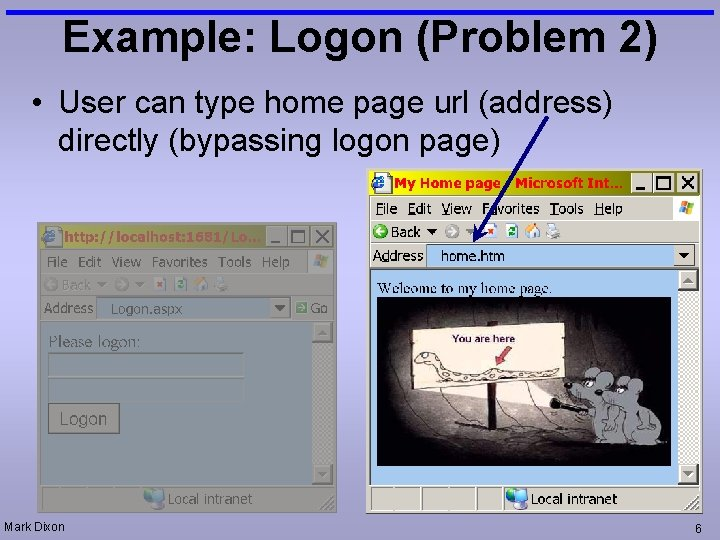Example: Logon (Problem 2) • User can type home page url (address) directly (bypassing logon page) Mark Dixon 6Solution • Need way for: – password page to tell home page – that user logged in OK Mark Dixon 7Technique: Dead-Drop Variables • 2 Spies wish to pass message between each other without actually meeting • Arrange a dead-drop location – one spy leaves message at location – other spy visits location later to pick up message • Variables used as dead-drop containers Mark Dixon 8Example: Logon v 3 (code) Logon 3. aspx Home 3. aspx <script language="VB" runat="server"> Dim Logon. OK As Boolean Sub Page_Load() Dim un As String Dim pw As String Logon. OK = False If Request. Form("btn. Logon") > "" Then un = txt. User. Name. Value pw = txt. Pass. Word. Value If un = "mark" And pw = "soft 131" Then Logon. OK = True Response. Redirect("home 3. htm") Else msg. inner. Text = "Login details incorrect. " End If End Sub </script> <html> <head><title></head> <body> <form runat="server"> Please logon: <input id="txt. User. Name" type="text" runat="server" /> <input id="txt. Pass. Word" type="text" runat="server" /> <input id="btn. Logon" type="submit" value="Logon" runat="server" /> <p id="msg" runat="server"></p> </form> </body> </html> Logon. OK True <script runat="server" language="VB"> Dim Logon. OK As Boolean Sub Page_Load() If Logon. OK = False Then Response. Redirect("Logon 3. aspx") End If End Sub </script> <html> <head><title>My Home page</title></head> <body> <p> Welcome to my home page. <img src="You. Are. Here. jpg" /> </p> </body> </html> Does not work: always redirect to logon Variables do not persist between pages Mark Dixon 9Example: Logon v 3 (Error) • Variables – don't persist between pages Mark Dixon 10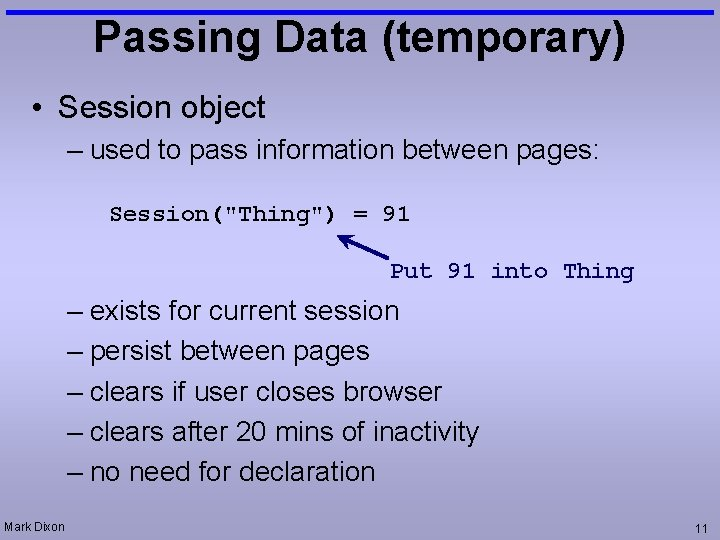Passing Data (temporary) • Session object – used to pass information between pages: Session("Thing") = 91 Put 91 into Thing – exists for current session – persist between pages – clears if user closes browser – clears after 20 mins of inactivity – no need for declaration Mark Dixon 11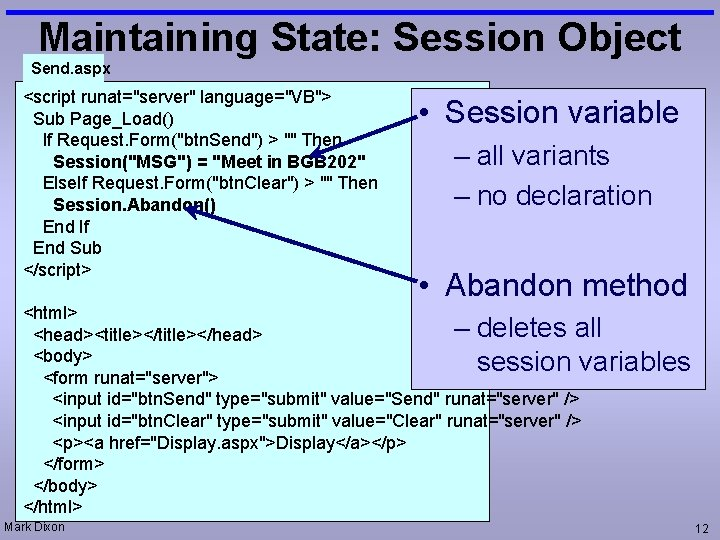Maintaining State: Session Object Send. aspx <script runat="server" language="VB"> Sub Page_Load() If Request. Form("btn. Send") > "" Then Session("MSG") = "Meet in BGB 202" Else. If Request. Form("btn. Clear") > "" Then Session. Abandon() End If End Sub </script> • Session variable – all variants – no declaration • Abandon method <html> – deletes all <head><title></head> <body> session variables <form runat="server"> <input id="btn. Send" type="submit" value="Send" runat="server" /> <input id="btn. Clear" type="submit" value="Clear" runat="server" /> <p><a href="Display. aspx">Display</a></p> </form> </body> </html> Mark Dixon 12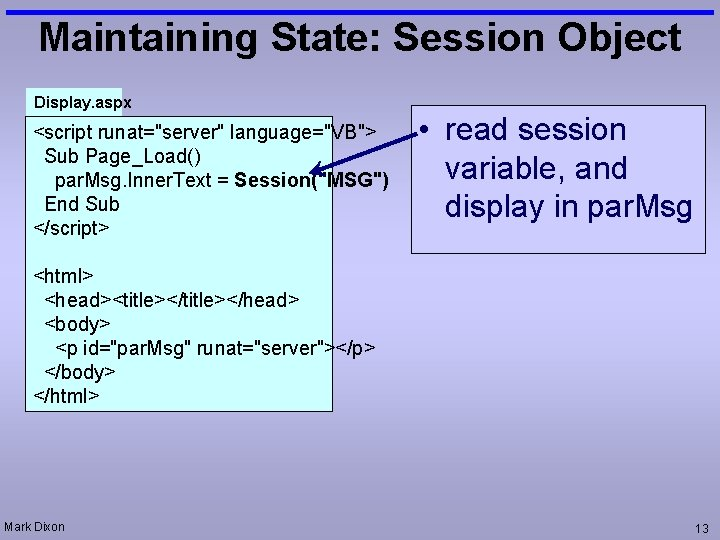Maintaining State: Session Object Display. aspx <script runat="server" language="VB"> Sub Page_Load() par. Msg. Inner. Text = Session("MSG") End Sub </script> • read session variable, and display in par. Msg <html> <head><title></head> <body> <p id="par. Msg" runat="server"></p> </body> </html> Mark Dixon 13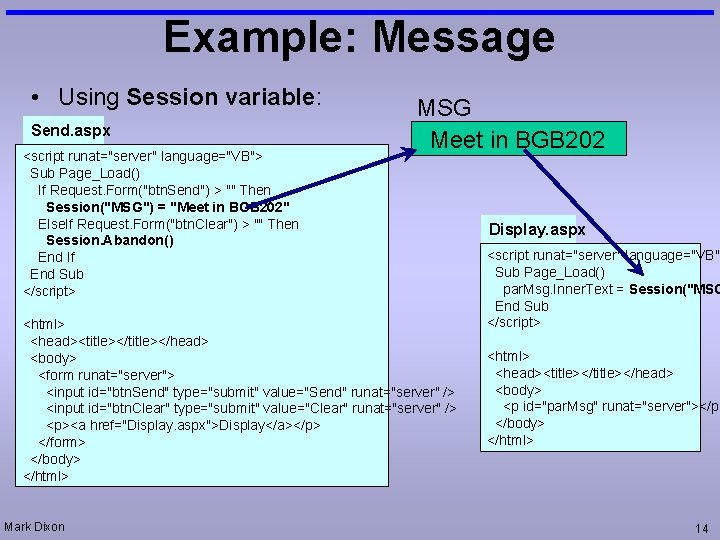Example: Message • Using Session variable: Send. aspx <script runat="server" language="VB"> Sub Page_Load() If Request. Form("btn. Send") > "" Then Session("MSG") = "Meet in BGB 202" Else. If Request. Form("btn. Clear") > "" Then Session. Abandon() End If End Sub </script> MSG Meet in BGB 202 <html> <head><title></head> <body> <form runat="server"> <input id="btn. Send" type="submit" value="Send" runat="server" /> <input id="btn. Clear" type="submit" value="Clear" runat="server" /> <p><a href="Display. aspx">Display</a></p> </form> </body> </html> Mark Dixon Display. aspx <script runat="server" language="VB"> Sub Page_Load() par. Msg. Inner. Text = Session("MSG End Sub </script> <html> <head><title></head> <body> <p id="par. Msg" runat="server"></p> </body> </html> 14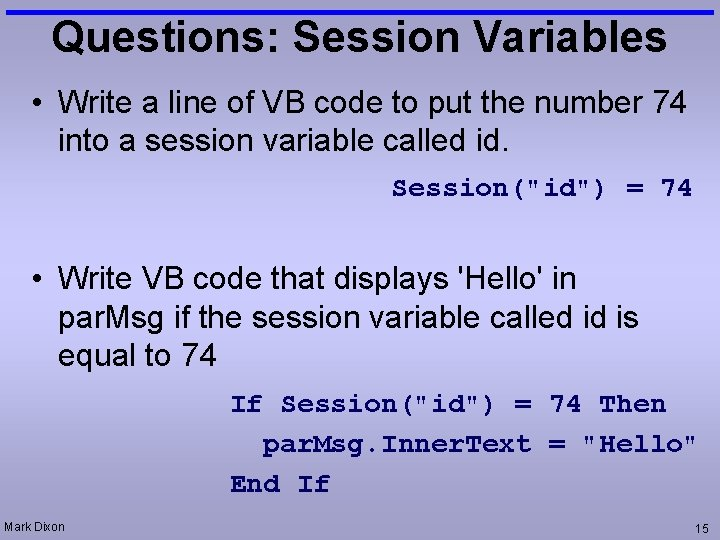Questions: Session Variables • Write a line of VB code to put the number 74 into a session variable called id. Session("id") = 74 • Write VB code that displays 'Hello' in par. Msg if the session variable called id is equal to 74 If Session("id") = 74 Then par. Msg. Inner. Text = "Hello" End If Mark Dixon 15Passing Data (temporary) • Query Strings – Useful for passing information between pages via links Mark Dixon 16Maintaining State: Query Strings • Data added to end of URL (address): http: //localhost/page. asp? Surname=Bob Query String • ASP code can use this data: – Request. Query. String("Surname") • would return the value "Bob" • Form method=get – data automatically added to query string Mark Dixon 17Example: Date-Time Menu. aspx <html> <head> </head> <body> <p>What background colour do you want for you date information? <a href=Date. Time. aspx? Colour=yellow>Yellow</a> <a href=Date. Time. aspx? Colour=cyan>Light Blue</a> </body> </html> Date. Time. aspx <html> <head> </head> <body bgcolor=<%=request. querystring("Colour")%>> <p>The date is <%=Format(Now(), "D")%>. <p>The time is <%=Format(Now(), "T")%>. </body> </html> Mark Dixon 18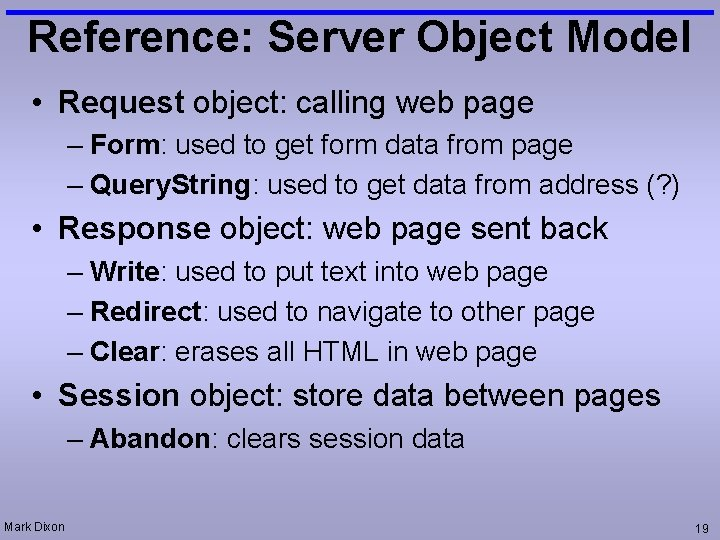Reference: Server Object Model • Request object: calling web page – Form: used to get form data from page – Query. String: used to get data from address (? ) • Response object: web page sent back – Write: used to put text into web page – Redirect: used to navigate to other page – Clear: erases all HTML in web page • Session object: store data between pages – Abandon: clears session data Mark Dixon 19Passing Data (persistent) • Cookies (not covered in this module) – stored on users’ (client) hard drive – persists between sessions • Database/file (covered in later lectures) – stored on server hard drive – persists between sessions Mark Dixon 20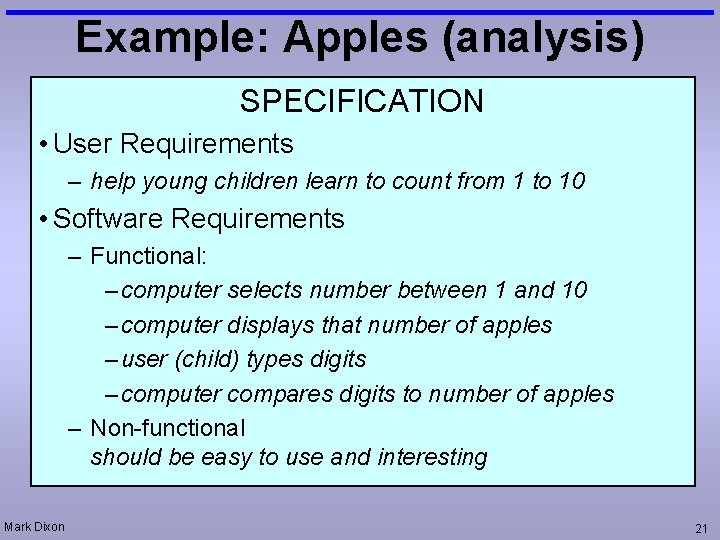Example: Apples (analysis) SPECIFICATION • User Requirements – help young children learn to count from 1 to 10 • Software Requirements – Functional: – computer selects number between 1 and 10 – computer displays that number of apples – user (child) types digits – computer compares digits to number of apples – Non-functional should be easy to use and interesting Mark Dixon 21Example: Apples v 2 (design) • Functionality: • • Mark Dixon computer selects number between 1 and 10 computer displays that number of apples user types digits computer compares digits to number of apples 22Data Representation • Stored data vs. display – display: seven images of apples (easier for people to understand) <img <img – Stored data: 7 (easier to process) Mark Dixon src=‘apple. gif src=‘apple. gif /> /> Dim num. Apples As Integer h = "" For a = 1 To num. Apples h = h & "<img src='Apple. gif'>" Next quest. Inner. Html = h 23Example: Apples v 2 (code) Apples. aspx Dim n As Long Sub Page_Load() Dim html As String Dim a As Long If Request. Form("btn. Start") > "" Then n = 1 + Int(Rnd() * 9) html = "How many apples are there? " For a = 1 To n html = html & "<img src=Apple. gif>" Next quest. Inner. Html = html Session("Num. Apples") = n msg. Inner. Html = "" txt. Ans. Value = "" btn. Start. Disabled = True btn. Check. Disabled = False Else. If Request. Form("btn. Check") > "" Then n = Session("Num. Apples") If CInt(txt. Ans. Value) = CInt(n) Then msg. Inner. Html = "Correct, well done!" Else msg. Inner. Html = "Sorry, please try again. " End If btn. Start. Disabled = False btn. Check. Disabled = True End If End Sub Mark Dixon Dim n As Long Sub Page_Load() Dim html As String Dim a As Long If Request. Form("btn. Start") > "" Then n = 1 + Int(Rnd() * 9) html = "How many apples are there? <b For a = 1 To n html = html & "<img src=Apple. gif>" Next quest. Inner. Html = html Session("Num. Apples") = n msg. Inner. Html = "" txt. Ans. Value = "" btn. Start. Disabled = True btn. Check. Disabled = False 24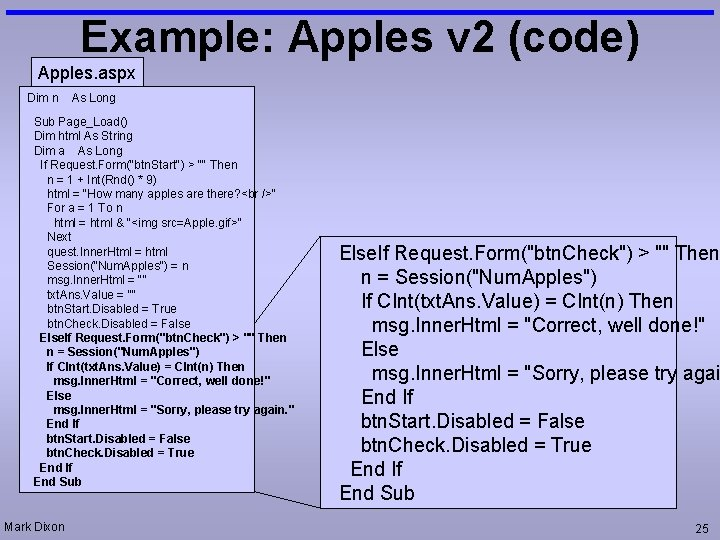Example: Apples v 2 (code) Apples. aspx Dim n As Long Sub Page_Load() Dim html As String Dim a As Long If Request. Form("btn. Start") > "" Then n = 1 + Int(Rnd() * 9) html = "How many apples are there? " For a = 1 To n html = html & "<img src=Apple. gif>" Next quest. Inner. Html = html Session("Num. Apples") = n msg. Inner. Html = "" txt. Ans. Value = "" btn. Start. Disabled = True btn. Check. Disabled = False Else. If Request. Form("btn. Check") > "" Then n = Session("Num. Apples") If CInt(txt. Ans. Value) = CInt(n) Then msg. Inner. Html = "Correct, well done!" Else msg. Inner. Html = "Sorry, please try again. " End If btn. Start. Disabled = False btn. Check. Disabled = True End If End Sub Mark Dixon Else. If Request. Form("btn. Check") > "" Then n = Session("Num. Apples") If CInt(txt. Ans. Value) = CInt(n) Then msg. Inner. Html = "Correct, well done!" Else msg. Inner. Html = "Sorry, please try agai End If btn. Start. Disabled = False btn. Check. Disabled = True End If End Sub 25Problem Solving Strategies • bottom-up – Create a detailed solution first – Then look for best solution – refactoring – process of: • changing internal design of code, • without altering what it does • top-down – plan overall design – fill in details in practice mixed – novices favour bot-up, experts top-down Mark Dixon 26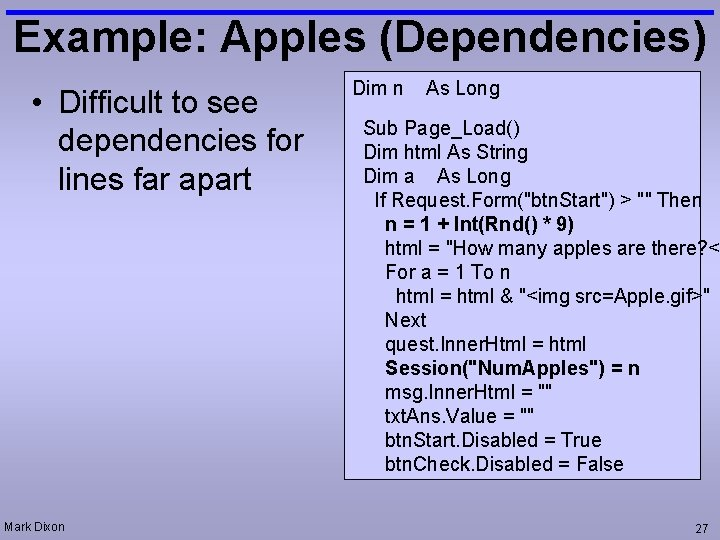Example: Apples (Dependencies) • Difficult to see dependencies for lines far apart Mark Dixon Dim n As Long Sub Page_Load() Dim html As String Dim a As Long If Request. Form("btn. Start") > "" Then n = 1 + Int(Rnd() * 9) html = "How many apples are there? <b For a = 1 To n html = html & "<img src=Apple. gif>" Next quest. Inner. Html = html Session("Num. Apples") = n msg. Inner. Html = "" txt. Ans. Value = "" btn. Start. Disabled = True btn. Check. Disabled = False 27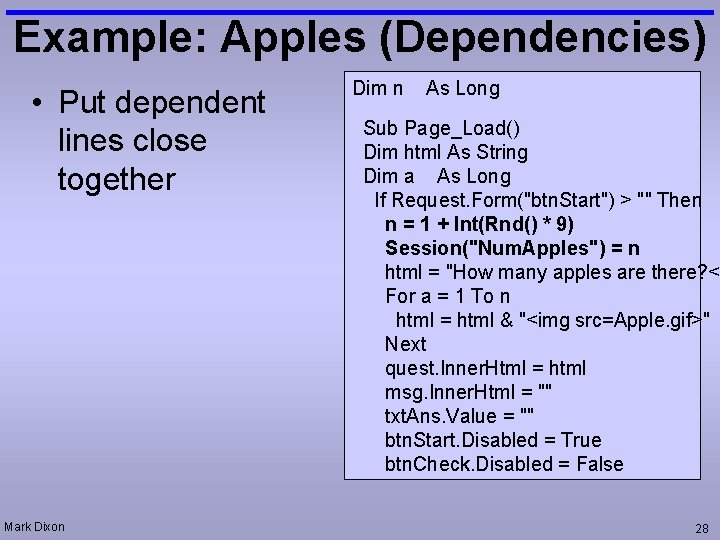Example: Apples (Dependencies) • Put dependent lines close together Mark Dixon Dim n As Long Sub Page_Load() Dim html As String Dim a As Long If Request. Form("btn. Start") > "" Then n = 1 + Int(Rnd() * 9) Session("Num. Apples") = n html = "How many apples are there? <b For a = 1 To n html = html & "<img src=Apple. gif>" Next quest. Inner. Html = html msg. Inner. Html = "" txt. Ans. Value = "" btn. Start. Disabled = True btn. Check. Disabled = False 28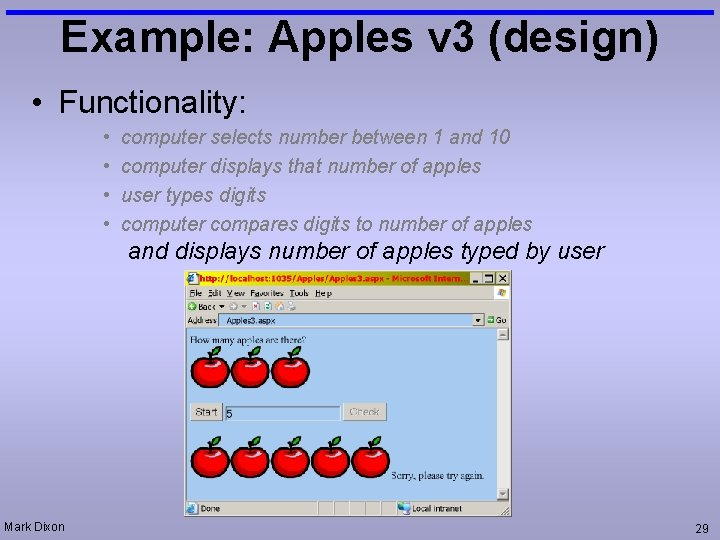Example: Apples v 3 (design) • Functionality: • • computer selects number between 1 and 10 computer displays that number of apples user types digits computer compares digits to number of apples and displays number of apples typed by user Mark Dixon 29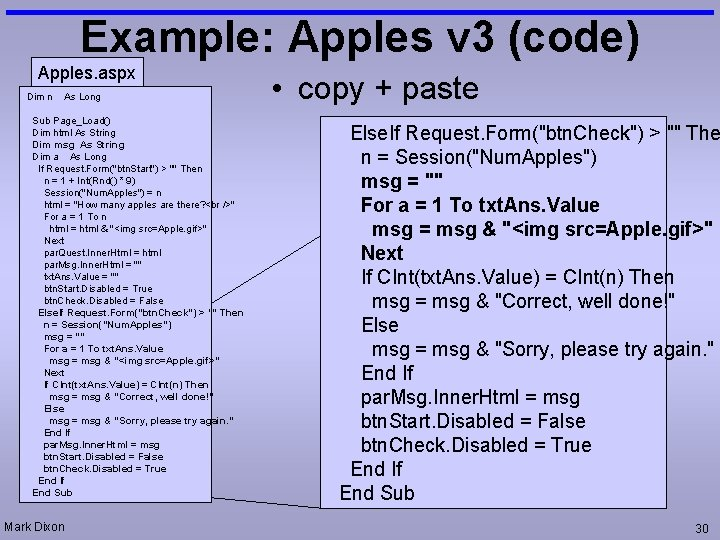Example: Apples v 3 (code) Apples. aspx Dim n As Long Sub Page_Load() Dim html As String Dim msg As String Dim a As Long If Request. Form("btn. Start") > "" Then n = 1 + Int(Rnd() * 9) Session("Num. Apples") = n html = "How many apples are there? " For a = 1 To n html = html & "<img src=Apple. gif>" Next par. Quest. Inner. Html = html par. Msg. Inner. Html = "" txt. Ans. Value = "" btn. Start. Disabled = True btn. Check. Disabled = False Else. If Request. Form("btn. Check") > "" Then n = Session("Num. Apples") msg = "" For a = 1 To txt. Ans. Value msg = msg & "<img src=Apple. gif>" Next If CInt(txt. Ans. Value) = CInt(n) Then msg = msg & "Correct, well done!" Else msg = msg & "Sorry, please try again. " End If par. Msg. Inner. Html = msg btn. Start. Disabled = False btn. Check. Disabled = True End If End Sub Mark Dixon • copy + paste Else. If Request. Form("btn. Check") > "" The n = Session("Num. Apples") msg = "" For a = 1 To txt. Ans. Value msg = msg & "<img src=Apple. gif>" Next If CInt(txt. Ans. Value) = CInt(n) Then msg = msg & "Correct, well done!" Else msg = msg & "Sorry, please try again. " End If par. Msg. Inner. Html = msg btn. Start. Disabled = False btn. Check. Disabled = True End If End Sub 30Modular Design • What do lines do (group summary)? Pick Num. of Apples Display Question Prepare for Response Mark Dixon n = 1 + Int(Rnd() * 9) Session("Num. Apples") = n html = "How many apples are there? < For a = 1 To n html = html & "<img src=Apple. gif>" Next par. Quest. Inner. Html = html par. Msg. Inner. Html = "" txt. Ans. Value = "" btn. Start. Disabled = True btn. Check. Disabled = False 31Modular Design (top level) • Top level reads like English algorithm: Dim n As Long Dim html As String Dim msg As String Dim a As Long Sub Page_Load() If Request. Form("btn. Start") > "" Then Pick. Random. Number. Of. Apples Display. Apples. Quest Prepare. For. Response Else. If Request. Form("btn. Check") > "" Then n = Session("Num. Apples") Display. Apples. User Display. Feedback Prepare. For. Quest End If End Sub Mark Dixon 32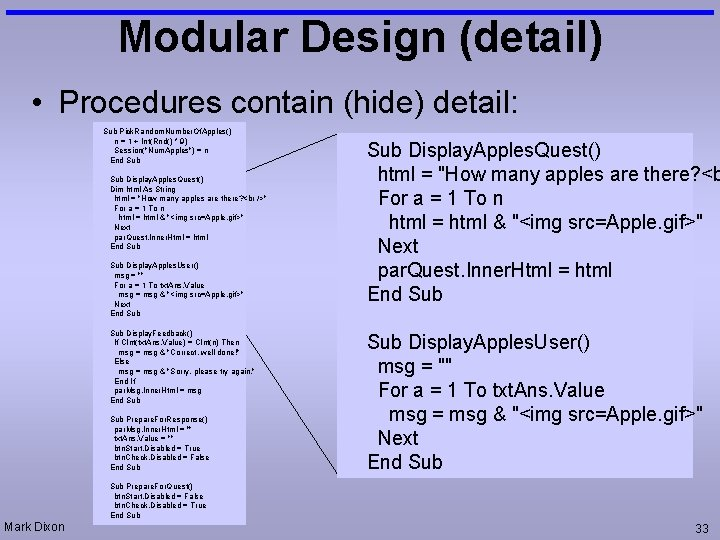Modular Design (detail) • Procedures contain (hide) detail: Sub Pick. Random. Number. Of. Apples() n = 1 + Int(Rnd() * 9) Session("Num. Apples") = n End Sub Display. Apples. Quest() Dim html As String html = "How many apples are there? " For a = 1 To n html = html & "<img src=Apple. gif>" Next par. Quest. Inner. Html = html End Sub Display. Apples. User() msg = "" For a = 1 To txt. Ans. Value msg = msg & "<img src=Apple. gif>" Next End Sub Display. Feedback() If CInt(txt. Ans. Value) = CInt(n) Then msg = msg & "Correct, well done!" Else msg = msg & "Sorry, please try again. " End If par. Msg. Inner. Html = msg End Sub Prepare. For. Response() par. Msg. Inner. Html = "" txt. Ans. Value = "" btn. Start. Disabled = True btn. Check. Disabled = False End Sub Display. Apples. Quest() html = "How many apples are there? <b For a = 1 To n html = html & "<img src=Apple. gif>" Next par. Quest. Inner. Html = html End Sub Display. Apples. User() msg = "" For a = 1 To txt. Ans. Value msg = msg & "<img src=Apple. gif>" Next End Sub Prepare. For. Quest() btn. Start. Disabled = False btn. Check. Disabled = True End Sub Mark Dixon 33Problem Solving: 9 dots • Join all 9 dots – with straight continuous lines Mark Dixon 34Problem Solving Process (Name Split) • Problem: a variable exists called n. This contains a person's full name (forename, then surname ). It needs to be split into two separate variables. Dim n As String n = "Ruth Jones" • Solution Process: – What do I do to solve this manually (on paper)? – How do I know where the forename ends and the surname begins? – The space is the key: • Find the space • everything before the space is the forename • everything after the space is the surname Mark Dixon 35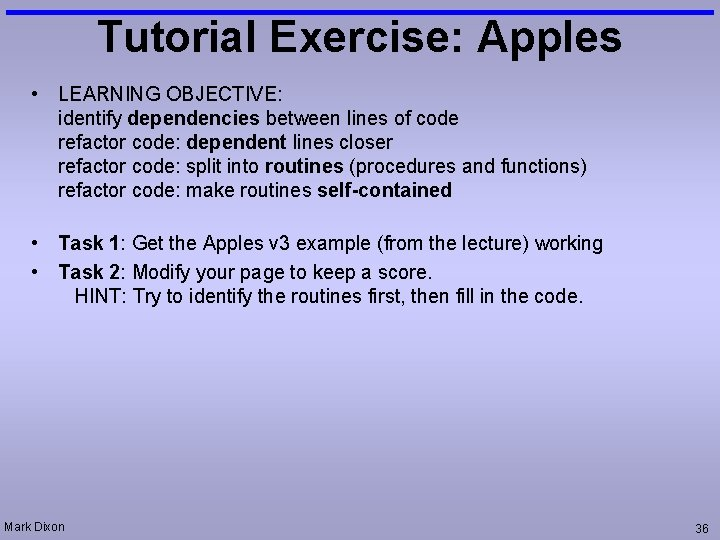Tutorial Exercise: Apples • LEARNING OBJECTIVE: identify dependencies between lines of code refactor code: dependent lines closer refactor code: split into routines (procedures and functions) refactor code: make routines self-contained • Task 1: Get the Apples v 3 example (from the lecture) working • Task 2: Modify your page to keep a score. HINT: Try to identify the routines first, then fill in the code. Mark Dixon 36Tutorial Exercise: Message • LEARNING OBJECTIVE: pass data between pages using session variables, and (form) selfposting • Task 1: Get the message example working (from the lecture) • Task 2: Change the send. aspx page so that when you click the buttons it gives some feedback as to what has happened. hint: add a paragraph Mark Dixon 37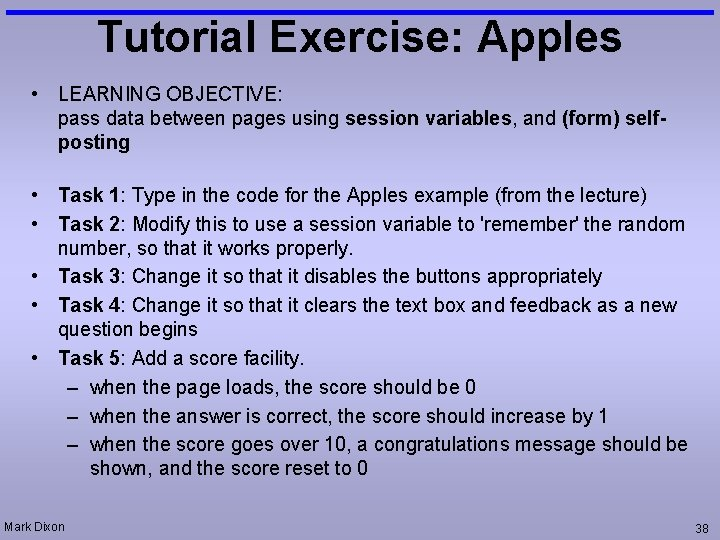Tutorial Exercise: Apples • LEARNING OBJECTIVE: pass data between pages using session variables, and (form) selfposting • Task 1: Type in the code for the Apples example (from the lecture) • Task 2: Modify this to use a session variable to 'remember' the random number, so that it works properly. • Task 3: Change it so that it disables the buttons appropriately • Task 4: Change it so that it clears the text box and feedback as a new question begins • Task 5: Add a score facility. – when the page loads, the score should be 0 – when the answer is correct, the score should increase by 1 – when the score goes over 10, a congratulations message should be shown, and the score reset to 0 Mark Dixon 38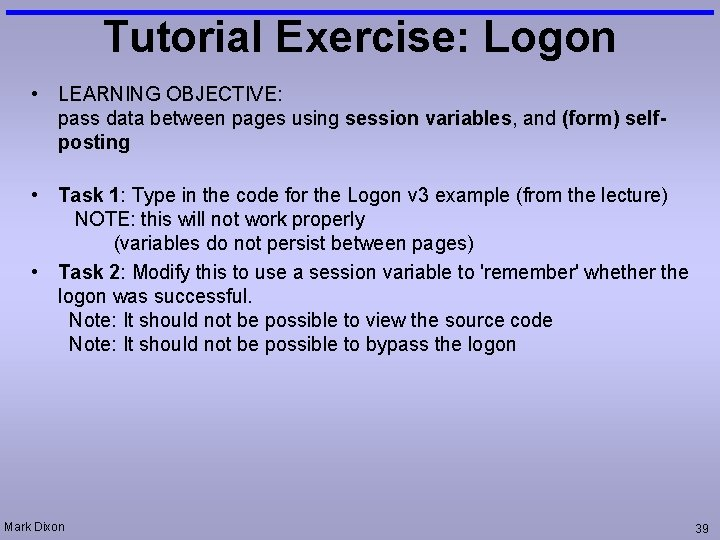Tutorial Exercise: Logon • LEARNING OBJECTIVE: pass data between pages using session variables, and (form) selfposting • Task 1: Type in the code for the Logon v 3 example (from the lecture) NOTE: this will not work properly (variables do not persist between pages) • Task 2: Modify this to use a session variable to 'remember' whether the logon was successful. Note: It should not be possible to view the source code Note: It should not be possible to bypass the logon Mark Dixon 39Tutorial Exercise: Date • LEARNING OBJECTIVE: pass data between pages using query strings • Task 1: Get the Date-Time example (from the lecture) working • Task 2: Modify your page to provide another choice of background colour. Mark Dixon 40